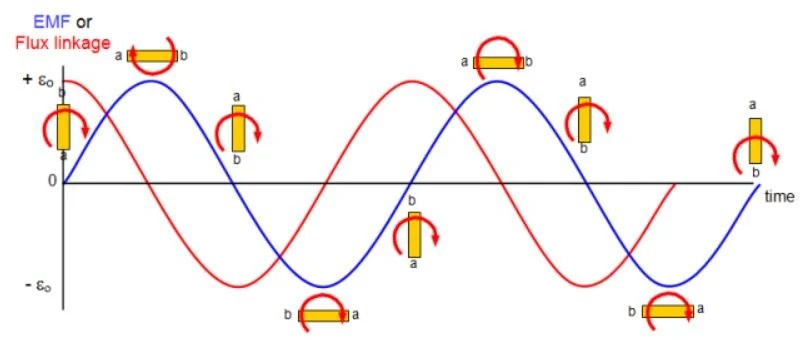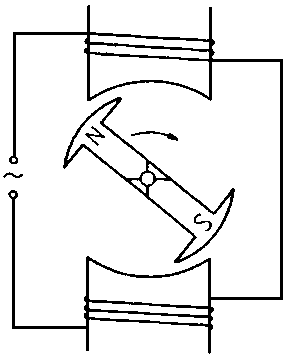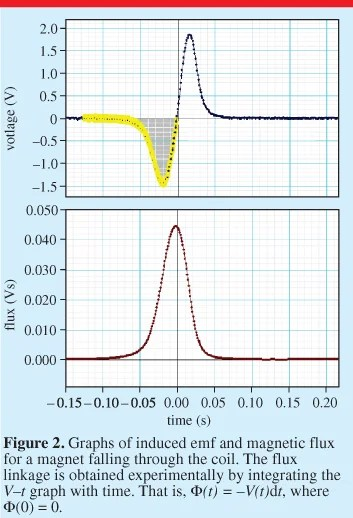# [Keywords] Electromagnetic Induction

### How to tell?

Mechanical movement generates electricity.

### Commonly used laws or rules:

Fleming’s Right Hand Rule (FRHR), Faraday’s Law and Lenz’s Law

### Commonly seen questions

Case study 1• Explain why when the coil rotates between two magnets, an emf (current) is induced in the coil.

As the coil (closed loop) rotates, there is a change in the magnetic flux linkage in the coil and according to Faraday’s law, an e.m.f will be induced in the coil (and hence an induced current in the coil).

Note: It is wrong to suggest that there is a magnetic flux linkage between the magnetic field and the coil. The magnetic flux linkage dealt only in the magnetic flux that was passing through the coil (that’s why the word linkage was used).

• The coil closer to N pole has induced current as shown by the red arrow. Explain how a current in this direction opposes the rotation of the coil.

Based on Fleming’s left-hand rule, when the current flows in the direction as shown (in the diagram, on the coil closer to the N pole), it will induce a magnetic field that will interact with the field by magnets. A downward directed force is created as a result. Since the original rotation of the coil is in clockwise direction (movement of the coil near N pole is going upward), the induced current in the direction shown creates a magnetic effect that opposes the rotation of the coil (a.k.a the change producing/causing it).

Note

If you just use Lenz’s law only to explain, you need to understand that the reason behind Lenz’s law is conservation of energy. And thus, the induced current will induce a magnetic field that interact with the field by magnets such that it does not cause the coil to speed up as that will violate the principle of conservation of energy.

• Explain why the induced e.m.f (current) is alternating. We will call the side of the coil closer to N pole (AB) and the side closer to S pole (CD) to make the explanation clearer.

Explanation for why there is an induced e.m.f –> above

Explanation why it will be alternating –> After half a revolution, side AB will now be at S pole while CD will be at N pole. Since arms AB and CD are moving in opposite direction to the starting position, the direction of induced e.m.f is also opposite to previously.

• Explain why the induced e.m.f will be varying.

When the plane of the coil is parallel to the magnetic field by the magnet, the coil cut across the magnetic field lines at the greatest rate. Since the rate of change of magnetic flux is maximum, the magnitude of induced e.m.f is maximum.

When the plane of the coil is perpendicular to the magnetic field by the magnet, the rate of change of magnetic flux is zero, the magnitude of induced e.m.f is minimum.

Note

Do remember that the change in magnetic flux –> there is induced e.m.f

But rate of change of magnetic flux –> determine the magnitude of the induced e.m.f

When e.m.f is zero, it is corresponding to maximum magnetic flux.Case Study 1A

Set-up in turbine or rotary parts to generate electricity. Just a slight modification to phrasing to suit the context to explain why e.m.f is induced in the coil.As the magnet(s) rotates, the magnetic fields setup by the magnets produce a continuous changing magnetic flux linkage in the coil wound on soft-iron core. By Faraday’s law, an e.m.f will be induced in the coil.

As the North pole of the magnet approaches and moves away from the coil, the induced e.m.f creates a North pole and South pole respectively in the coil to oppose the change producing it, according to Lenz’s law. This happens when the South pole of the magnet approaches and moves away from the coil as well. This alternating of polarities of the coils means that the induced e.m.f/current is alternating.

Case study 2Graphs of the magnetic flux and induced e.m.f in the coil against time as the magnet falls through the coil.

To get the e.m.f graph, you simply need to differentiate/ find the gradient of the magnetic flux graph. Do not forget that the answer (gradient) need to be reverse because of Lenz’s law.Other possible arrangement to show electromagnetic induction